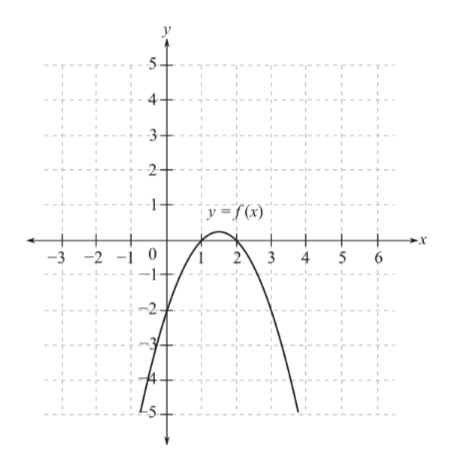### October 2021 archive

In my precalc 11 this week, we learned about the quadratic formula. This is another way to solve quadratic equations, other than factoring or completing the square. The quadratic formula looks like this:It is easy to solve an equation using the quadratic formula because all you do is put in the numbers, and use algebra to find the variable. To do this, you must find out what numbers in the polynomial are A, B and C.  A is always the number with the x², B is always with the x, and C is always the last number, without a variable. Remember that when there is no number with an x, there is an invisible 1. Here are some examples of what A, B, and C are in the following polynomials.This is how to solve a polynomial using the quadratic formula:In the next equation, the quadratic formula is used, but the answers are 2 rational roots. This shows that the equation could have been factored from the start, rather than doing the quadratic formula, which sometimes takes longer. When you need to solve a quadratic equation, first check if the numbers can be factored, or if you can use the technique of completing the square. If not, using the quadratic formula will guarantee the correct answer.In my precalc 11 class this week, I learned about graphing, and how it can relate to a quadratic equation or function. A quadratic function is in the form y=ax² + bx + c

These are all quadratic equations: Even if they don’t look like it at the start, they can be moved around into the correct form.These graphs in curved shapes are called parabolas:When an equation creates a graph in a parabola, it is a quadratic function. Parabolas have a vertex, which is either the highest or lowest point. If it is the highest point on the graph, it is called the maximum point. If it is at the bottom, it is the minimum point. Here are some examples:This week in precalc 11, I learned how to factor a polynomial, specifically when there is a fraction within it. Here are some examples of polynomials with fractions, that can be factored:To be able to factor these easily, there should be only integers with the variables. This means you must find a greatest common denominator (GCD), so all the terms have the same number at the bottom of the fraction. Then, the fraction can be removed as a greatest common factor (GCF), and the polynomial can be factored. Here are the above examples, with the steps to take to factor:

Remember, if there is a variable by itself without a number in front, there is always an invisible 1. Also, don’t forget that all numbers/variables are fractions, even if they aren’t shown that way at first. If a variable, like x, is not written as a fraction, it has a denominator of 1.You can also verify your answer when you are done, to check if it is correct. To do this, just take the final answer and use the distributive property with FOIL to get back to the polynomial you started with.

In week 5 of precalc 11, we started learning about factoring. This is a continuation of grade 10, but new factoring skills are being added on. One concept we learned about is factoring with a greatest common factor (GCF). This is when you remove a common factor from all the terms, so it becomes easier to factor. The GCF has to be something that is present in ALL terms. Remember, a variable, like x, can also be a part of the common factor. Here are some examples of binomials with a GCF:When you remove the GCF from a binomial or trinomial to factor, make sure to remove the same GCF from all terms. If there is a variable like x in two of the terms but not the other, then it cannot be part of the GCF that gets removed. For example:Finally, here are some more examples of how to remove the GCF from a binomial or trinomial. When you do this, the GCF is written in front, and the remaining the numbers are inside brackets. Sometimes this is all you do to simplify the question, but make sure to check if you can factor any further.

In my precalc 11 class this week, I learned how to rationalize a denominator. This happens when there is a fraction with a square root on the bottom. For example:When fractions are being simplified, it is better to show them with an integer on the bottom. If the denominator is not an integer, but a radical, you can rationalize it. To make the denominator a rational number, or a non-radical, multiply the whole fraction by 1. This is done my multiplying it by the radical on the bottom, that you want to get rid of. (Since the radical is on the top and bottom of a fraction it equals 1).

These fractions below are all equal to 1, because the numerator and denominator are the same:When you multiply the two fractions, make sure to multiply the radicals only with other radicals, and integers in front of the radicals (if it’s a mixed radical) with other integers. If there is no number in front of a radical, pretend there is a 1. Make sure not to multiply a radical with an integer! Here are a few examples of how to rationalize the denominator: